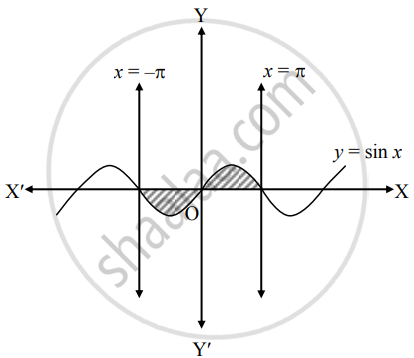# Find the area of the region bounded by the curve y = sin x, the X−axis and the given lines x = − π, x = π - Mathematics and Statistics

Sum

Find the area of the region bounded by the curve y = sin x, the X−axis and the given lines x = − π, x = π

#### Solution

Let A be the required area.

Consider the equation y = sin x∴ A = int_(-pi)^pi y  "d"x

= int_(-pi)^pi sin x  "d"x

= |int_(-pi)^0 sin x  "d"x| + int_0^pi sin x  "d"x

= |[- cos x]_(-pi)^0| + [- cos x]_0^pi

= | – [cos 0 – cos (– π)]| – (cos π – cos 0)

= | – [1 – (–1)] | – (–1 – 1)

= |– 2| + 2

= 2 + 2

= 4 sq.units

Concept: Area Bounded by the Curve, Axis and Line
Is there an error in this question or solution?